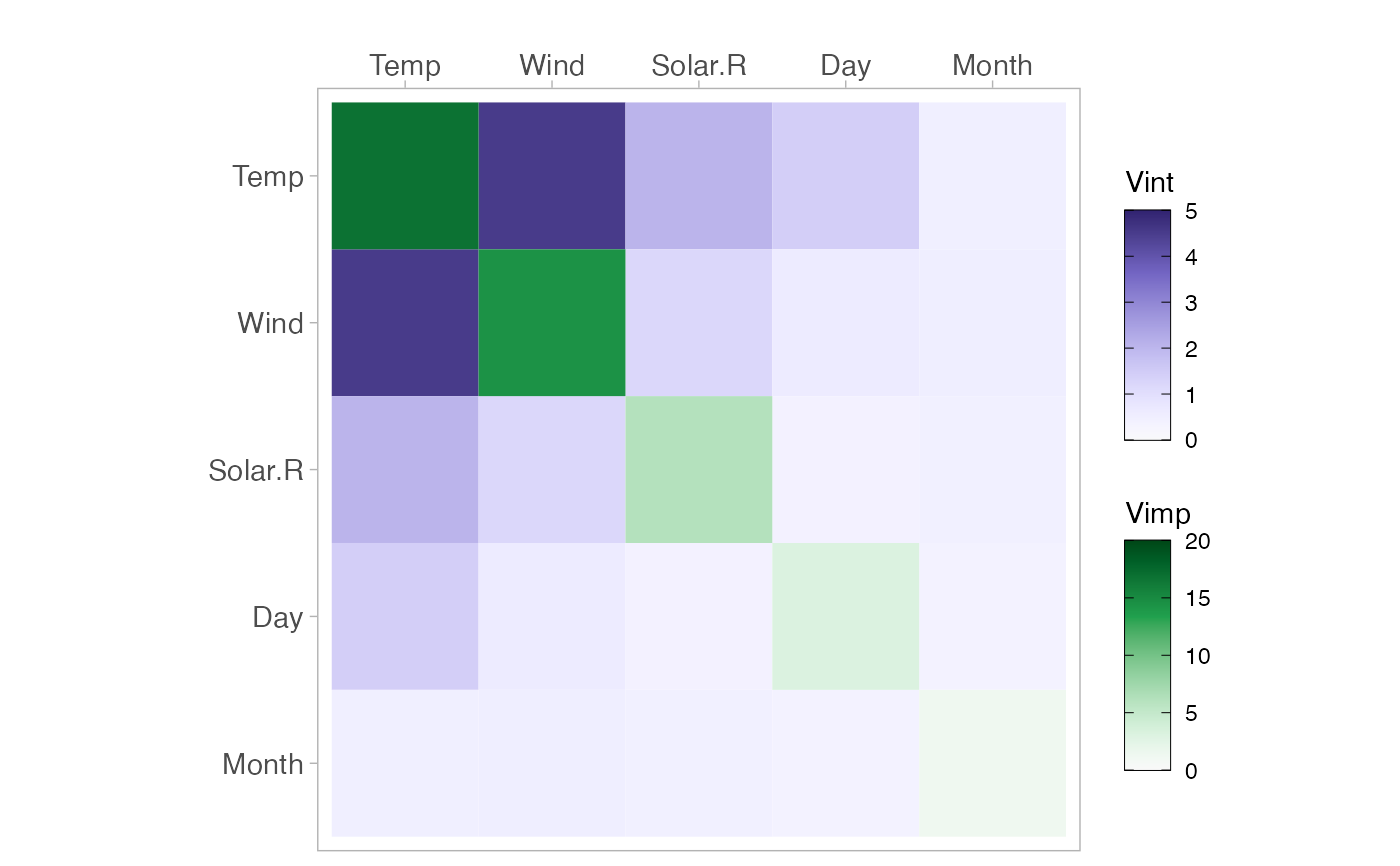Plots a Heatmap showing variable importance on the diagonal and variable interaction on the off-diagonal.

viviHeatmap(
mat,
intPal = rev(colorspace::sequential_hcl(palette = "Purples 3", n = 100)),
impPal = rev(colorspace::sequential_hcl(palette = "Greens 3", n = 100)),
intLims = NULL,
impLims = NULL,
border = FALSE,
angle = 0
)

## Arguments

mat A matrix, such as that returned by vivi, of values to be plotted. A vector of colours to show interactions, for use with scale_fill_gradientn. A vector of colours to show importance, for use with scale_fill_gradientn. Specifies the fit range for the color map for interaction strength. Specifies the fit range for the color map for importance. Logical. If TRUE then draw a black border around the diagonal elements. The angle to rotate the x-axis labels. Defaults to zero.

## Value

A heatmap plot showing variable importance on the diagonal and variable interaction on the off-diagonal.

## Examples

# \donttest{
library(ranger)
aq <- na.omit(airquality)
rF <- ranger(Ozone ~ ., data = aq, importance = "permutation")
myMat <- vivi(fit = rF, data = aq, response = "Ozone")
#> Agnostic variable importance method used.
#> Calculating interactions...
viviHeatmap(myMat)# }